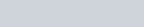Words with or without
Question:

Words with or without meaning are to be formed using all the letters of the word EXAMINATION. The probability that the letter $M$ appears at the fourth position in any such word is:

1. $\frac{1}{66}$

2. $\frac{1}{11}$

3. $\frac{1}{9}$

4. $\frac{2}{11}$

Correct Option: , 2

Solution:

AAEIIMNNOTXTotal words with $\mathrm{M}$ at fourth Place $=\frac{10 !}{2 ! 2 ! 2 !}$

Total words $=\frac{11 !}{2 ! 2 ! 2 !}$

Required probability $=\frac{10 !}{11 !}=\frac{1}{11}$